# Introduction¶

Based on data from YFCC100m dataset, this Notebook explores a processing example for visualizing frequentation patterns in a 100x100km Grid (worldwide). The data used here was converted from YFCC CSVs to the raw lbsn structure using lbsntransform package.

Our goal was to illustrate a complete typical visualization pipeline, from reading data to processing to visualization. There're additional steps included such as archiving intermediate results or creating an alternative interactive visualization.

This is the second notebook in a series of four notebooks:

In this Notebook, we describe a complete visualization pipeline, exploring worldwide frequentation patterns from YFCC dataset based on a 100x100km grid. The following steps are some of the parts explained:

• get data from LBSN raw db (PostgreSQL select)
• store raw data to CSV, load from CSV
• create a parametrized world-wide grid
• implement a binary search for fast mapping of coordinates to grid-bins
• perform the bin-assignment with actual coordinates from Flickr YFCC dataset
• chunk processing into smaller parts, to reduce memory load
• summarize different metrics for bins: postcount, usercount, userdays
• create methods to reduce from individual code parts
• measure timing of different steps, to compare processing time with hll-dataset approach
• load and store intermediate results from and to *.pickle and *.CSV

System requirements

The raw notebook requires about 16 GB of Memory, the hll notebook about 8 GB.

Use Shift+Enter to walk through the Notebook

# Preparations¶

## Parameters¶

This is a collection of parameters that affect processing and graphics.

In :
from pathlib import Path

GRID_SIZE_METERS = 100000 # the size of grid cells in meters
# (spatial accuracy of worldwide measurement)
# process x number of hll records per chunk.
# Increasing this number will consume more memory,
# but reduce processing time because less SQL queries
# are needed.
CHUNK_SIZE = 5000000
# target projection: Mollweide (epsg code)
EPSG_CODE = 54009
# note: Mollweide defined by _esri_
# in epsg.io's database
CRS_PROJ = f"esri:{EPSG_CODE}"
# Input projection (Web Mercator)
CRS_WGS = "epsg:4326"
# define path to output directory (figures etc.)
OUTPUT = Path.cwd().parents / "out"


Load all dependencies at once, as a means to verify that everything required to run this notebook is available.

In :
import os
import csv
import sys
import math
import colorcet
import psycopg2
import holoviews as hv
import mapclassify as mc
import geopandas as gp
import pandas as pd
import numpy as np
import matplotlib.pyplot as plt
from pathlib import Path
from typing import List, Tuple, Dict, Union, Generator, Optional
from pyproj import Transformer, CRS, Proj
from geoviews import opts
from shapely.geometry import shape, Point, Polygon
from shapely.ops import transform
from cartopy import crs
from matplotlib import colors
from IPython.display import clear_output, display, HTML, Markdown
# optionally, enable shapely.speedups
# which makes some of the spatial
# queries running faster
import shapely.speedups as speedups
speedups.enable()


Load helper module from ../py/module/tools.py. This also allows to import code from other jupyter notebooks, synced to *.py with jupytext.

In :
module_path = str(Path.cwd().parents / "py")
if module_path not in sys.path:
sys.path.append(module_path)
from modules import tools, preparations


Set pandas colwidth.

In :
pd.set_option('display.max_colwidth', 25)


Activate autoreload of changed python files:

In :
%load_ext autoreload


Load memory profiler extension

In :
%load_ext memory_profiler


Plot used package versions for future use:

•••
package xarray Shapely pyproj pandas numpy matplotlib mapclassify ipython holoviews geoviews geopandas Fiona Cartopy bokeh
version 0.16.1 1.7.1 2.6.1.post1 1.1.2 1.19.1 3.3.2 2.3.0 7.18.1 1.13.4 1.8.1 0.8.1 1.8.17 0.18.0 2.2.1

### Connect to database¶

Password is loaded from .env file specified in container setup hlldb.

The docker stack contains a full backup of the YFCC database converted to the privacy-aware datastructure. In this Notebook, we're only working with a small part of the data from the table spatial.latlng.

Define credentials as environment variables

In :
db_user = "postgres"
# set connection variables
db_host = "rawdb"
db_port = "5432"
db_name = "rawdb"


Connect to empty Postgres database running HLL Extension. Note that only readonly privileges are needed.

is defined as a global variable, for simplicity, to make it available in all functions.

In :
db_connection = psycopg2.connect(
host=db_host,
port=db_port,
dbname=db_name,
user=db_user,
)


Test connection:

In :
db_query = """
SELECT 1;
"""
# create pandas DataFrame from database data
df = pd.read_sql_query(db_query, db_connection)

?column?
0 1

For simplicity, the db connection parameters and query are stored in a class:

In :
db_conn = tools.DbConn(db_connection)
db_conn.query("SELECT 1")

Out:
?column?
0 1

## LBSN structure data introduction¶

The Location Based Social Network (LBSN) structure was developed as a standardized conceptual data model for analyzing, comparing and relating information of different LBSN in visual analytics research and beyond. The primary goal is to systematically characterize LBSN data aspects in a common scheme that enables privacy-by-design for connected software, data handling and information visualization.

Modular design

The core lbsn structure is described in a platform independent Protocol Buffers file. The Proto file can be used to compile and implement the proposed structure in any language such as Python, Java or C++.

This structure is tightly coupled with a relational datascheme (Postgres SQL) that is maintained separately, inluding a privacy-aware version that can be used for visualization purposes. The database is ready to use with several provided Docker containers that optionally include a PGadmin interface.

A documentation of the LBSN structure components is available at https://lbsn.vgiscience.org/structure/.

First, some statistics for the data we're working with.

Note that the following two queries take about 10 Minutes each. They're not necessary to run the notebook.
In :
%%time
db_query = """
SELECT count(*) FROM topical.post;
"""

display(Markdown(
f"There're "
f"<strong style='color: red;'>"
f"{db_conn.query(db_query)['count']:,.0f}</strong> "
f"distinct records (Flickr photo posts) in this table."))


There're 100,000,000 distinct records (Flickr photo posts) in this table.

CPU times: user 24.5 ms, sys: 18.2 ms, total: 42.6 ms
Wall time: 6min 31s


The Flickt YFCC 100M dataset includes 99,206,564 photos and 793,436 videos from 581,099 different photographers, and 48,469,829 of those are geotagged .

Photos are available in schema topical and table post.

With a query get_stats_query defined in tools module, we can get a more fine grained output of statistics for this table:

In :
%%time
db_query = tools.get_stats_query("topical.post")
stats_df = db_conn.query(db_query)
stats_df["bytes/ct"] = stats_df["bytes/ct"].fillna(0).astype('int64')
display(stats_df)

metric bytes/ct bytes_pretty bytes_per_row
0 core_relation_size 48428793856 45 GB 484.0
1 visibility_map 0 0 bytes 0.0
2 free_space_map 11919360 11 MB 0.0
3 table_size_incl_toast 49340284928 46 GB 493.0
4 indexes_size 26507223040 25 GB 265.0
5 total_size_incl_toast_and_indexes 75847507968 71 GB 758.0
6 live_rows_in_text_representation 42719824906 40 GB 427.0
7 ------------------------------ 0 None NaN
8 row_count 100000000 None NaN
9 live_tuples 0 None NaN
10 dead_tuples 0 None NaN
CPU times: user 60.7 ms, sys: 41.3 ms, total: 102 ms
Wall time: 13min 37s


Data structure preview (get random 10 records):

In :
db_query = "SELECT * FROM topical.post WHERE post_geoaccuracy != 'unknown' LIMIT 5;"
first_10_df = db_conn.query(db_query)
display(first_10_df)

origin_id post_guid post_latlng place_guid city_guid country_guid user_guid post_publish_date post_body post_geoaccuracy ... post_url post_type post_filter post_quote_count post_share_count post_language input_source user_mentions modified post_content_license
0 2 6985418911 0101000020E61000009F02603C837354C0198D7C5EF18C... 56558566 12772085 56043648 39089491@N00 2012-03-15 20:41:23 None place ... http://www.flickr.com/photos/39089491@N00/6985... image None None None None None [] 2020-02-15 05:09:05.922172 3
1 2 10201275523 0101000020E6100000494DBB9866D753C08BF9B9A129D3... 55855853 12697691 56043697 55289779@N00 2013-10-11 06:10:28 None latlng ... http://www.flickr.com/photos/55289779@N00/1020... image None None None None None [] 2020-02-15 05:09:05.922172 5
2 2 7289030198 0101000020E6100000C7D79E59127F52C0A7ECF483BA5E... 28751381 12761347 56043648 53430201@N03 2012-05-28 21:26:39 None latlng ... http://www.flickr.com/photos/53430201@N03/7289... image None None None None None [] 2020-02-15 05:09:05.922172 3
3 2 4572998878 0101000020E6100000EC1516DC0FC351C0E544BB0A292B... 2496012 12758726 56043648 78969707@N00 2010-05-03 01:37:20 Greeny's, Christines, Andy, Jeeane and Lou's p... latlng ... http://www.flickr.com/photos/78969707@N00/4572... image None None None None None [] 2020-02-15 05:09:05.922172 3
4 2 3973434963 0101000020E6100000D20149D8B793D8BFD670917BBABC... 20220221 20080321 56043644 71322403@N00 2009-10-02 11:04:00 None latlng ... http://www.flickr.com/photos/71322403@N00/3973... image None None None None None [] 2020-02-15 05:09:05.922172 1

5 rows × 28 columns

### Get data from db and write to CSV¶

To speed up processing in this notebook, we're going to work on a CSV file instead of live data retrieved from the database. The yfcc raw db contains many attributes, for the visualization and metrics used in this notebook, we only need the following attributes:

• latitude and longitude coordinates of geotagged yfcc photos, to bin coordinates to the grid and counting number of posts
• the user_guid, to count distinct users
• the date of photo creation, to count distinct userdays
To make processing of raw data comparable to hll data processing, we're also reducing the accuracy of lat/lng coordinates with a GeoHash Precision of 5 to about 4 km spatial accuracy. Similarly, we reduce temporal granularity to dates, because time is not needed for measuring userdays. Such considerations benefit both privacy and ease of processing.
In :
def get_yfccposts_fromdb(
chunk_size: int = CHUNK_SIZE) -> List[pd.DataFrame]:
"""Returns YFCC posts from db"""
sql = f"""
SELECT  ST_Y(ST_PointFromGeoHash(ST_GeoHash(t1.post_latlng, 5), 5)) As "latitude",
ST_X(ST_PointFromGeoHash(ST_GeoHash(t1.post_latlng, 5), 5)) As "longitude",
t1.user_guid,
to_char(t1.post_create_date, 'yyyy-MM-dd') As "post_create_date"
FROM topical.post t1
WHERE
NOT ((ST_Y(t1.post_latlng) = 0) AND (ST_X(t1.post_latlng) = 0))
AND
t1.post_geoaccuracy IN ('place', 'latlng', 'city');
"""
# execute query, enable chunked return
return pd.read_sql(sql, con=db_connection, chunksize=chunk_size)

def write_chunkeddf_tocsv(
filename: str, usecols: List[str], chunked_df: List[pd.DataFrame],
chunk_size: int = CHUNK_SIZE, output: Path = OUTPUT):
"""Write chunked dataframe to CSV"""
for ix, chunk_df in enumerate(chunked_df):
mode = 'a'
if ix == 0:
mode = 'w'
chunk_df.to_csv(
output / "csv" / filename,
mode=mode, columns=usecols,
clear_output(wait=True)
display(
f'Stored {(ix*chunk_size)+len(chunk_df)} '
f'posts to CSV..')


The sql explained:

SELECT  ST_Y(ST_PointFromGeoHash(ST_GeoHash(t1.post_latlng, 5), 5)) As "latitude",  -- lat and long coordinates from
ST_X(ST_PointFromGeoHash(ST_GeoHash(t1.post_latlng, 5), 5)) As "longitude", -- PostGis geometry, with GeoHash 5
t1.user_guid,                                                    -- the user_guid from Flickr (yfcc100m)
to_char(t1.post_create_date, 'yyyy-MM-dd') As "post_create_date" -- the photo's date of creation, without time,
-- to count distinct days
FROM topical.post t1                                                     -- the table reference from lbsn raw:
-- scheme (facet) = "topical", table = "post"
WHERE
NOT ((ST_Y(t1.post_latlng) = 0) AND (ST_X(t1.post_latlng) = 0))          -- excluding Null Island
AND
t1.post_geoaccuracy IN ('place', 'latlng', 'city');                      -- lbsn raw geoaccuracy classes,
-- equals Flickr geoaccuracy levels 8-16*


* The maximum resolution for maps will be 50 or 100km raster, therefore 8 (==city in lbsn raw structure) appears to be a reasonable choice. Also see Flickr yfcc to raw lbsn mapping

Execute query:

In :
%%time
filename = "yfcc_posts.csv"
usecols = ["latitude", "longitude", "user_guid", "post_create_date"]
if Path(OUTPUT / "csv" / filename).exists():
print(f"CSV already exists, skipping load from db..")
else:
write_chunkeddf_tocsv(
chunked_df=get_yfccposts_fromdb(),
filename=filename,
usecols=usecols)

'Stored 47843599 posts to CSV..'
CPU times: user 7min 45s, sys: 38 s, total: 8min 23s
Wall time: 18min 55s


RAW file size:

In :
raw_size_mb = Path(OUTPUT / "csv" / "yfcc_posts.csv").stat().st_size / (1024*1024)
print(f"Size: {raw_size_mb:.2f} MB")

Size: 2494.16 MB


### RAW Questions¶

To anticipate some questions or assumptions:

Why do I need a DB connection to get yfcc data, the original yfcc files are available as CSV?

YFCC original CSVs are formatted in a custom format. LBSN raw structure offers a systematic data scheme for handling of Social Media data such as yfcc. The database also allows us to better illustrate how to limit the query to only the data that is needed.

## Create Grid¶

1. Define Mollweide crs string for pyproj/Proj4 and WGS1984 for Social Media imports
In :
# define Transformer ahead of time
# with xy-order of coordinates
PROJ_TRANSFORMER = Transformer.from_crs(
CRS_WGS, CRS_PROJ, always_xy=True)

# also define reverse projection
PROJ_TRANSFORMER_BACK = Transformer.from_crs(
CRS_PROJ, CRS_WGS, always_xy=True)

1. create bounds from WGS1984 and project to Mollweide
In :
XMIN = PROJ_TRANSFORMER.transform(
-180, 0)
XMAX = PROJ_TRANSFORMER.transform(
180, 0)
YMAX = PROJ_TRANSFORMER.transform(
0, 90)
YMIN = PROJ_TRANSFORMER.transform(
0, -90)

In :
print(f'Projected bounds: {[XMIN, YMIN, XMAX, YMAX]}')

Projected bounds: [-18040095.696147293, -9020047.848073646, 18040095.696147293, 9020047.848073646]

1. Create 100x100 km (e.g.) Grid
In :
def create_grid_df(
grid_size: int = GRID_SIZE_METERS,
xmin: float = XMIN,
ymin: float = YMIN,
xmax: float = XMAX,
ymax: float = YMAX,
report: bool = None,
return_rows_cols: bool = None):
"""Creates dataframe polygon grid based on width and length in Meters"""
width = grid_size
length = grid_size
cols = list(range(int(np.floor(xmin)), int(np.ceil(xmax)), width))
rows = list(range(int(np.floor(ymin)), int(np.ceil(ymax)), length))
if report:
print(len(cols))
print(len(rows))
rows.reverse()

polygons = []
for x in cols:
for y in rows:
# combine to tuple: (x,y, poly)
# and append to list
polygons.append(
(x, y,
Polygon([
(x, y),
(x+width, y),
(x+width, y-length),
(x, y-length)])))
# create a pandas dataframe
# from list of tuples
grid = pd.DataFrame(polygons)
# name columns
col_labels=['xbin', 'ybin', 'bin_poly']
grid.columns = col_labels
# use x and y as index columns
grid.set_index(['xbin', 'ybin'], inplace=True)
if return_rows_cols:
return grid, rows, cols
return grid

In :
grid, rows, cols = create_grid_df(
report=True, return_rows_cols=True)

361
181

In :
grid.head()

Out:
bin_poly
xbin ybin
-18040096 8979952 POLYGON ((-18040096 8...
8879952 POLYGON ((-18040096 8...
8779952 POLYGON ((-18040096 8...
8679952 POLYGON ((-18040096 8...
8579952 POLYGON ((-18040096 8...

Create a geodataframe from dataframe:

In :
def grid_to_gdf(
grid: pd.DataFrame, crs_proj: str = CRS_PROJ) -> gp.GeoDataFrame:
"""Convert grid pandas DataFrame to geopandas Geodataframe"""
grid = gp.GeoDataFrame(
grid.drop(
columns=["bin_poly"]),
geometry=grid.bin_poly)
grid.crs = crs_proj
return grid

In :
grid = grid_to_gdf(grid)


Add columns for aggregation

In :
def reset_metrics(
grid: gp.GeoDataFrame,
metrics: List[str] = ["postcount", "usercount", "userdays"], setzero: bool = None):
"""Remove columns from GeoDataFrame and optionally fill with 0"""
for metric in metrics:
try:
grid.drop(metric, axis=1, inplace=True)
grid.drop(f'{metric}_cat', axis=1, inplace=True)
except KeyError:
pass
if setzero:
grid.loc[:, metric] = 0

In :
reset_metrics(grid)
display(grid)

geometry
xbin ybin
-18040096 8979952 POLYGON ((-18040096.0...
8879952 POLYGON ((-18040096.0...
8779952 POLYGON ((-18040096.0...
8679952 POLYGON ((-18040096.0...
8579952 POLYGON ((-18040096.0...
... ... ...
17959904 -8620048 POLYGON ((17959904.00...
-8720048 POLYGON ((17959904.00...
-8820048 POLYGON ((17959904.00...
-8920048 POLYGON ((17959904.00...
-9020048 POLYGON ((17959904.00...

65341 rows × 1 columns

Read World geometries data

In :
%%time
world = gp.read_file(gp.datasets.get_path('naturalearth_lowres'), crs=CRS_WGS)
world = world.to_crs(CRS_PROJ)

CPU times: user 354 ms, sys: 15.9 ms, total: 370 ms
Wall time: 368 ms


### Preview Grid¶

In :
base = grid.plot(figsize=(22,28), color='white', edgecolor='black', linewidth=0.1)
# combine with world geometry
plot = world.plot(ax=base)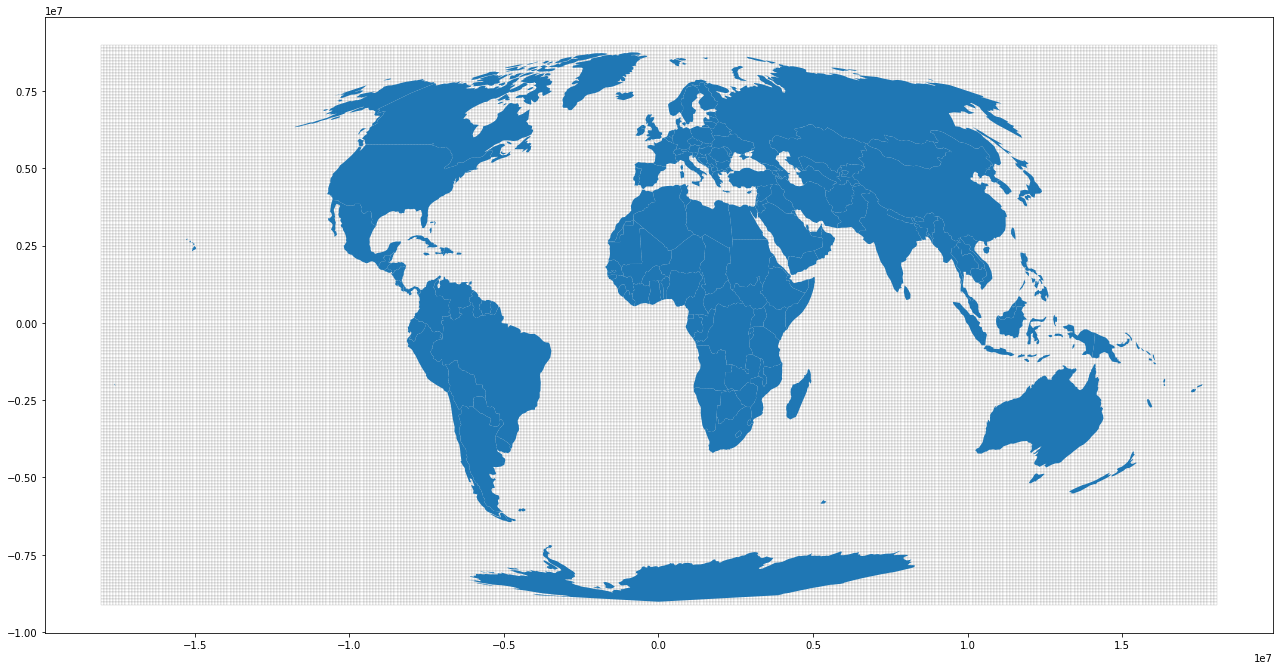# Prepare binary search¶

The aggregation speed is important here and we should not use polygon intersection. Since we're working with a regular grid and floating point numbers, a binary search is likely one of the fastest ways for our context. numpy.digitize provides a binary search, but it must be adapted to for the spatial context. A lat or lng value is assigned to the nearest bin matching. We get our lat and lng bins from our original Mollweide grid, which are regularly spaced at 100km interval. Note that we need to do two binary searches, for lat and for lng values.

## Create test points¶

In :
testpoint = Point(8.546377, 47.392323)
testpoint2 = Point(13.726359, 51.028512)
gdf_testpoints = gp.GeoSeries([testpoint, testpoint2], crs=CRS_WGS)
# project geometries to Mollweide
gdf_testpoints_proj = gdf_testpoints.to_crs(CRS_PROJ)

In :
gdf_testpoints_proj.x

Out:
671646.5840955656

Preview map for testpoint

In :
base = world.plot(figsize=(22,28), color='white', edgecolor='black', linewidth=0.1)
plot = gdf_testpoints_proj.plot(ax=base)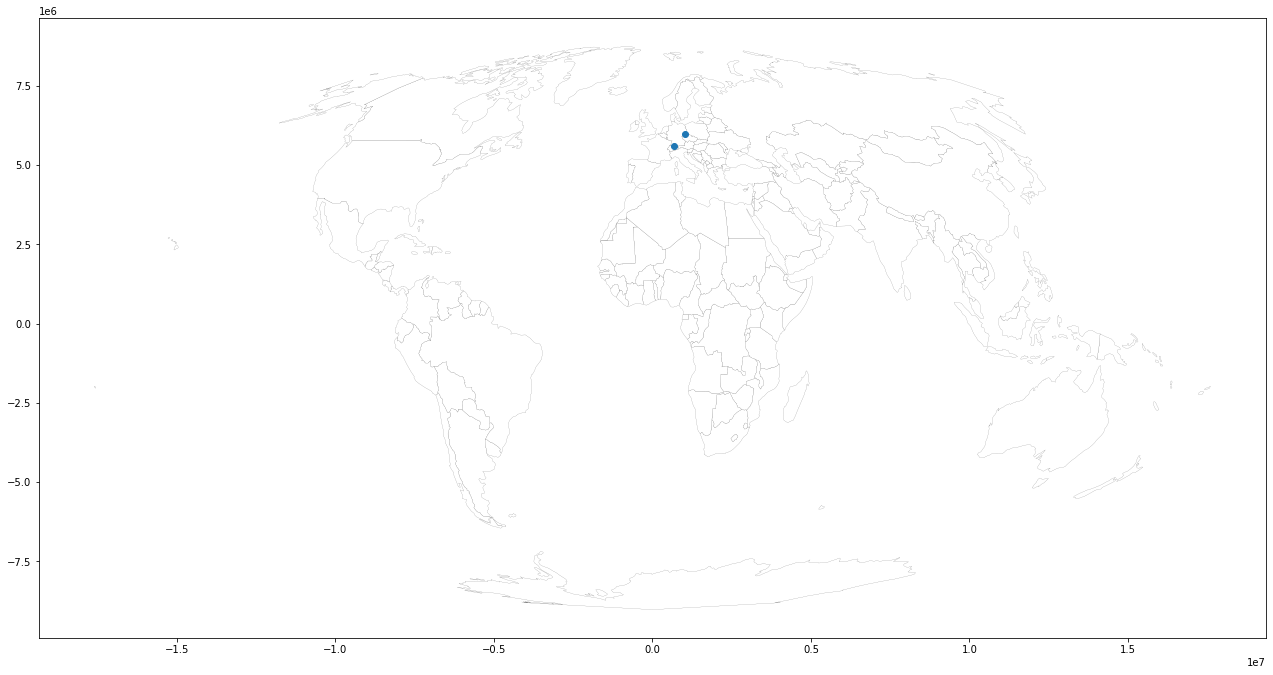### Use np.digitize() to assign coordinates to the grid¶

np.digitize is implemented in terms of np.searchsorted. This means that a binary search is used to bin the values, which scales much better for larger number of bins than the previous linear search. It also removes the requirement for the input array to be 1-dimensional.

Create 2 bins for each axis of existing Mollweide rows/cols grid:

In :
ybins = np.array(rows)
xbins = np.array(cols)


Create 2 lists with a single entry (testpoint coordinate)

In :
test_point_list_x = np.array([gdf_testpoints_proj.x, gdf_testpoints_proj.x])
test_point_list_y = np.array([gdf_testpoints_proj.y, gdf_testpoints_proj.y])


Find the nearest bin for x coordinate (returns the bin-index):

In :
x_bin = np.digitize(test_point_list_x, xbins) - 1
display(x_bin)

array([187, 190])

Check value of bin (the y coordinate) based on returned index:

In :
testpoint_xbin_idx = xbins[[x_bin, x_bin]]
display(testpoint_xbin_idx)

array([659904, 959904])

Repeat the same for y-testpoint:

In :
y_bin = np.digitize(test_point_list_y, ybins) - 1
display(y_bin)

array([33, 29])
In :
testpoint_ybin_idx = ybins[[y_bin, y_bin]]
display(testpoint_ybin_idx)

array([5679952, 6079952])

➡️ 759904 / 5579952 and 1059904 / 5979952 are indexes that we can use in our geodataframe index to return the matching grid-poly for each point

### Highlight Testpoint in Grid¶

Get grid-poly by index from testpoint

In :
grid.loc[testpoint_xbin_idx, testpoint_ybin_idx]

Out:
geometry    POLYGON ((659904.000 ...
Name: (659904, 5679952), dtype: geometry

Convert shapely bin poly to Geoseries and plot

In :
testpoint_grids = gp.GeoSeries(
[grid.loc[testpoint_xbin_idx, testpoint_ybin_idx].geometry, grid.loc[testpoint_xbin_idx, testpoint_ybin_idx].geometry])
testpoint_grids.plot()

Out:
<AxesSubplot:>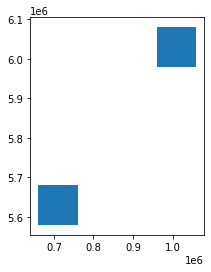### Preview map with testpoint and assigned bin¶

Set auto zoom with buffer:

In :
minx, miny, maxx, maxy = testpoint_grids.total_bounds
buf = 1000000

In :
# a figure with a 1x1 grid of Axes
fig, ax = plt.subplots(1, 1,figsize=(10,8))
ax.set_xlim(minx-buf, maxx+buf)
ax.set_ylim(miny-buf, maxy+buf)
base = world.plot(ax=ax, color='white', edgecolor='black', linewidth=0.1)
grid_base = testpoint_grids.plot(ax=base, facecolor='red', linewidth=0.1)
plot = gdf_testpoints_proj.plot(ax=grid_base, markersize=8, color='blue')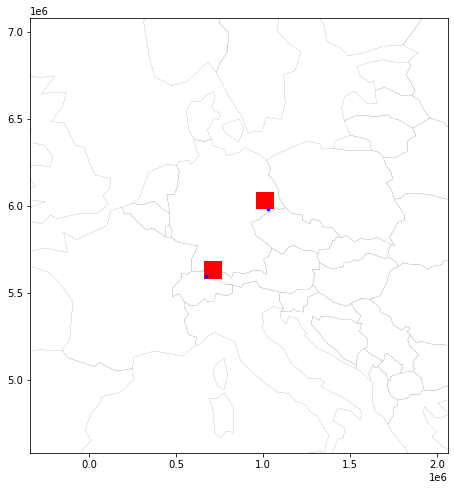## Prepare functions¶

Now that it has been visually verified that the algorithm works, lets create functions for the main processing job.

In :
def get_best_bins(
search_values_x: np.array, search_values_y: np.array,
xbins: np.array, ybins: np.array) -> Tuple[np.ndarray, np.ndarray]:
"""Will return best bin for a lat and lng input

Note: prepare bins and values in correct matching projection

Args:
search_values_y: A list of projected latitude values
search_values_x: A list of projected longitude values
xbins: 1-d array of bins to snap lat/lng values
ybins: 1-d array of bins to snap lat/lng values

Returns:
Tuple[int, int]: A list of tuples with 2 index positions for the best
matching bins for each lat/lng
"""
xbins_idx = np.digitize(search_values_x, xbins, right=False)
ybins_idx = np.digitize(search_values_y, ybins, right=False)
return (xbins[xbins_idx-1], ybins[ybins_idx-1])


Create xbins and ybins directly, as a means to supporting import *

In :
_, ROWS, COLS = create_grid_df(return_rows_cols=True)
YBINS = np.array(ROWS)
XBINS = np.array(COLS)


## Test with LBSN data¶

We're going to test the binning of coordinates on a part of the YFCC geotagged images.

Prepare lat/lng tuple of lower left corner and upper right corner to crop sample map:

In :
# Part of Italy and Sicily
bbox_italy = (
7.8662109375, 36.24427318493909,
19.31396484375, 43.29320031385282)
bbox = bbox_italy


Calculate bounding box with 1000 km buffer. For that, project the bounding Box to Mollweide, apply the buffer, and project back to WGS1984:

In :
# convert to Mollweide
minx, miny = PROJ_TRANSFORMER.transform(
bbox_italy, bbox_italy)
maxx, maxy = PROJ_TRANSFORMER.transform(
bbox_italy, bbox_italy)
# apply buffer and convetr back to WGS1984
min_buf = PROJ_TRANSFORMER_BACK.transform(minx-buf, miny-buf)
max_buf = PROJ_TRANSFORMER_BACK.transform(maxx+buf, maxy+buf)
bbox_italy_buf = [min_buf, min_buf, max_buf, max_buf]


Select columns and types for improving speed

In :
usecols = ['latitude', 'longitude']
dtypes = {'latitude': float, 'longitude': float}
reset_metrics(grid)


In :
%%time
OUTPUT / "csv" / "yfcc_posts.csv", usecols=usecols, dtype=dtypes, encoding='utf-8')
print(len(df))

47843599
CPU times: user 35.7 s, sys: 7.99 s, total: 43.7 s
Wall time: 43.7 s


Filter on bounding box (Italy)

In :
def filter_df_bbox(
df: pd.DataFrame, bbox: Tuple[float, float, float, float],
inplace: bool = True):
"""Filter dataframe with bbox on latitude and longitude column"""
df.query(
f'({bbox} < longitude) & '
f'(longitude <  {bbox}) & '
f'({bbox} < latitude) & '
f'(latitude < {bbox})',
inplace=True)
# set index to asc integers
if inplace:
df.reset_index(inplace=True, drop=True)
return
return df.reset_index(inplace=False, drop=True)


Execute and count number of posts in the bounding box:

In :
%%time
filter_df_bbox(df=df, bbox=bbox_italy_buf)
print(f"There're {len(df):,.0f} YFCC geotagged posts located within the bounding box.")

There're 13,361,348 YFCC geotagged posts located within the bounding box.

latitude longitude
0 39.484863 -0.373535
1 45.417480 12.326660
2 48.142090 11.579590
3 51.130371 4.328613
4 52.448730 -1.560059
CPU times: user 1.49 s, sys: 701 ms, total: 2.19 s
Wall time: 2.19 s


### Project coordinates to Mollweide¶

Projection speed can be increased by using a predefined pyproj.Transformer. We're also splitting our input-dataframe into a list of dataframe, each containing 1 Million records, so we can process the data in chunks.

In :
def proj_df(df, proj_transformer: Transformer = PROJ_TRANSFORMER):
"""Project pandas dataframe latitude and longitude decimal degrees
using predefined proj_transformer"""
if 'longitude' not in df.columns:
return
xx, yy = proj_transformer.transform(
df['longitude'].values, df['latitude'].values)
# assign projected coordinates to
# new columns x and y
# the ':' means: replace all values in-place
df.loc[:, "x"] = xx
df.loc[:, "y"] = yy
# Drop WGS coordinates
df.drop(columns=['longitude', 'latitude'], inplace=True)

In :
%%time
proj_df(df)
print(f'Projected {len(df.values):,.0f} coordinates')

Projected 13,361,348 coordinates

x y
0 -31872.166464 4.731738e+06
1 991046.755221 5.385474e+06
2 901798.894258 5.677544e+06
3 324299.385019 5.991327e+06
4 -114739.576009 6.127444e+06
CPU times: user 10.9 s, sys: 477 ms, total: 11.4 s
Wall time: 11.4 s


### Perform the bin assignment¶

In :
%%time
xbins_match, ybins_match = get_best_bins(
search_values_x=df['x'].to_numpy(),
search_values_y=df['y'].to_numpy(),
xbins=xbins, ybins=ybins)

CPU times: user 2.43 s, sys: 176 ms, total: 2.61 s
Wall time: 2.6 s

In :
len(xbins_match)

Out:
13361348
In :
xbins_match[:10]

Out:
array([ -40096,  959904,  859904,  259904, -140096,  759904,  559904,
-40096,  -40096,  159904])
In :
ybins_match[:10]

Out:
array([4779952, 5479952, 5679952, 6079952, 6179952, 5279952, 5979952,
5979952, 6079952, 4979952])

## A: Post Count per grid¶

Attach target bins to original dataframe. The : means: modify all values in-place

In :
df.loc[:, 'xbins_match'] = xbins_match
df.loc[:, 'ybins_match'] = ybins_match
# set new index column
df.set_index(['xbins_match', 'ybins_match'], inplace=True)
# drop x and y columns not needed anymore
df.drop(columns=['x', 'y'], inplace=True)

In :
df.head()

Out:
xbins_match ybins_match
-40096 4779952
959904 5479952
859904 5679952
259904 6079952
-140096 6179952

Count per bin. Since we know that there are no duplicates in the YFCC100M dataset, we can take a shortcut and simply count the size of the index.

In :
%%time
cardinality_series = df.groupby(
df.index).size()

CPU times: user 21.6 s, sys: 2.3 s, total: 23.9 s
Wall time: 23.8 s

In :
cardinality_series.index = pd.MultiIndex.from_tuples(
cardinality_series.index, names=['xbin', 'ybin'])

In :
cardinality_series.head()

Out:
xbin     ybin
-340096  3879952       3
4179952       1
4279952     967
4379952       1
4479952    6433
dtype: int64
In :
reset_metrics(grid, ["postcount"], setzero=True)


Append Series with calculated counts to grid (as new column) based on index match:

In :
grid.loc[cardinality_series.index, 'postcount'] = cardinality_series

In :
grid[grid["postcount"] > 0].head()

Out:
geometry postcount
xbin ybin
-340096 6179952 POLYGON ((-340096.000... 771
6079952 POLYGON ((-340096.000... 12990
5979952 POLYGON ((-340096.000... 5075
5879952 POLYGON ((-340096.000... 1
5779952 POLYGON ((-340096.000... 1521

### Preview post count map¶

Use headtail_breaks classification scheme because it is specifically suited to map long tailed data, see Jiang 2013

• Jiang, B. (August 01, 2013). Head/Tail Breaks: A New Classification Scheme for Data with a Heavy-Tailed Distribution. The Professional Geographer, 65, 3, 482-494.
In :
# global legend font size setting
plt.rc('legend', **{'fontsize': 16})

In :
def leg_format(leg):
"Format matplotlib legend entries"
for lbl in leg.get_texts():
label_text = lbl.get_text()
lower = label_text.split(",").lstrip("[(")
upper = label_text.split(",").rstrip(")]")
new_text = f'{float(lower):,.0f} - {float(upper):,.0f}'
lbl.set_text(new_text)

def title_savefig_mod(
title, save_fig, grid_size_meters: int = GRID_SIZE_METERS):
"""Update title/output name if grid size is not 100km"""
if grid_size_meters == 100000:
return title, save_fig
km_size = grid_size_meters/1000
title = f'{title} ({km_size:.0f}km grid)'
if save_fig:
save_fig = save_fig.replace(
'.png', f'_{km_size:.0f}km.png')
return title, save_fig

def save_plot(
grid: gp.GeoDataFrame, title: str, column: str, save_fig: str = None,
output: Path = OUTPUT, bbox: Tuple[float, float, float, float] = None,
proj_transformer: Transformer = PROJ_TRANSFORMER, buf: int = 1000000,
world = None):
"""Plot GeoDataFrame with matplotlib backend, optionaly export as png"""
fig, ax = plt.subplots(1, 1,figsize=(10,12))
if bbox is not None:
# create bounds from WGS1984 italy and project to Mollweide
minx, miny = proj_transformer.transform(
bbox, bbox)
maxx, maxy = proj_transformer.transform(
bbox, bbox)
ax.set_xlim(minx-buf, maxx+buf)
ax.set_ylim(miny-buf, maxy+buf)
title, save_fig = title_savefig_mod(
title, save_fig)
ax.set_title(title, fontsize=20)
base = grid.plot(
ax=ax, column=column, cmap='OrRd', scheme='headtail_breaks',
legend=True, legend_kwds={'loc': 'lower right'})
if world is None:
gp.datasets.get_path('naturalearth_lowres'), crs=CRS_WGS)
world = world.to_crs(CRS_PROJ)
# combine with world geometry
plot = world.plot(
ax=base, color='none', edgecolor='black', linewidth=0.1)
leg = ax.get_legend()
leg_format(leg)
if not save_fig:
return
fig.savefig(output / "figures" / save_fig, dpi=300, format='PNG',

In :
save_plot(
grid=grid, title='Post Count',
column='postcount', save_fig='postcount_sample.png',
bbox=bbox_italy, world=world)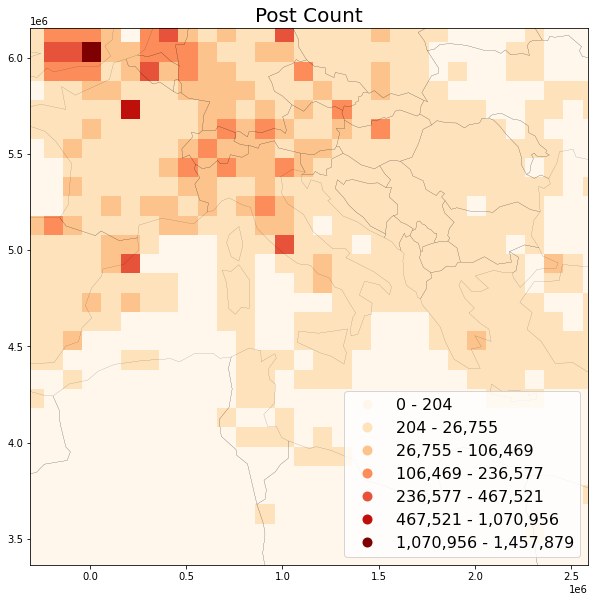## B: User Count per grid¶

When using RAW data, the caveat for calculating usercounts is that all distinct ids per bin must be present first, before calculating the total count. Since the input data (Social Media posts) is spatially unordered, this requires either a two-pass approach (e.g. writing intermediate data to disk and performing the count in a second pass), or storing all user guids per bin in-memory. We're using the second approach here.

What can be done to reduce memory load is to process the input data in chunks. After each chunk has been processed, Python's garbage collection can do its work and remove everything that is not needed anymore.

Furthermore, we can store intermediate data to CSV, which is also more efficient than loading data from DB.

These ideas are combined in the methods below. Adjust default chunk_size of 5000000 to your needs.

Specify input data

First, specify the columns that need to be retrieved from the database. In addition to lat and lng, we need the user_guid for calculating usercounts.

In :
usecols = ['latitude', 'longitude', 'user_guid']


Adjust method for stream-reading from CSV in chunks:

In :
%%time
OUTPUT / "csv" / "yfcc_posts.csv", usecols=usecols, iterator=True,
dtype=dtypes, encoding='utf-8', chunksize=CHUNK_SIZE)

CPU times: user 2.22 ms, sys: 3.82 ms, total: 6.04 ms
Wall time: 5.19 ms

In :
def proj_report(df, cnt, inplace: bool = False):
"""Project df with progress report"""
proj_df(df)
clear_output(wait=True)
print(f'Projected {cnt:,.0f} coordinates')
if inplace:
return
return df

In :
%%time
# filter
chunked_df = [
filter_df_bbox(
df=chunk_df, bbox=bbox_italy_buf, inplace=False)
for chunk_df in iter_csv]

# project
projected_cnt = 0
for chunk_df in chunked_df:
projected_cnt += len(chunk_df)
proj_report(
chunk_df, projected_cnt, inplace=True)


Projected 13,361,348 coordinates

user_guid x y
0 71322403@N00 -31872.166464 4.731738e+06
1 22841923@N02 991046.755221 5.385474e+06
2 25622716@N02 901798.894258 5.677544e+06
3 84351449@N00 324299.385019 5.991327e+06
4 15181848@N02 -114739.576009 6.127444e+06
CPU times: user 1min 18s, sys: 4.46 s, total: 1min 23s
Wall time: 1min 23s


### Perform the bin assignment and count distinct users¶

First assign coordinates to bin using our binary search:

In :
def bin_coordinates(
df: pd.DataFrame, xbins:
np.ndarray, ybins: np.ndarray) -> pd.DataFrame:
"""Bin coordinates using binary search and append to df as new index"""
xbins_match, ybins_match = get_best_bins(
search_values_x=df['x'].to_numpy(),
search_values_y=df['y'].to_numpy(),
xbins=xbins, ybins=ybins)
# append target bins to original dataframe
# use .loc to avoid chained indexing
df.loc[:, 'xbins_match'] = xbins_match
df.loc[:, 'ybins_match'] = ybins_match
# drop x and y columns not needed anymore
df.drop(columns=['x', 'y'], inplace=True)

In :
def bin_chunked_coordinates(
chunked_df: List[pd.DataFrame], xbins:
np.ndarray = XBINS, ybins: np.ndarray = YBINS):
"""Bin coordinates of chunked dataframe"""
binned_cnt = 0
for ix, df in enumerate(chunked_df):
bin_coordinates(df, xbins, ybins)
df.set_index(['xbins_match', 'ybins_match'], inplace=True)
clear_output(wait=True)
binned_cnt += len(df)
print(f"Binned {binned_cnt:,.0f} coordinates..")

In :
%%time
bin_chunked_coordinates(chunked_df)

Binned 13,361,348 coordinates..

user_guid
xbins_match ybins_match
-40096 4779952 71322403@N00
959904 5479952 22841923@N02
859904 5679952 25622716@N02
259904 6079952 84351449@N00
-140096 6179952 15181848@N02
CPU times: user 6.98 s, sys: 317 ms, total: 7.29 s
Wall time: 7.19 s


Now group user_guids per bin in distinct sets. The demonstration below is based the first chunk of posts ():

In :
%%time
df = chunked_df
series_grouped = df["user_guid"].groupby(
df.index).apply(set)

CPU times: user 4.72 s, sys: 531 ms, total: 5.25 s
Wall time: 5.13 s

Out:
(-340096, 4279952)    {75315636@N00, 785589...
(-340096, 4479952)    {75315636@N00, 140161...
(-340096, 4579952)    {75315636@N00, 140161...
(-340096, 4679952)    {75315636@N00, 280791...
(-340096, 4779952)    {7183730@N06, 1871975...
Name: user_guid, dtype: object

Now we have sets of user_guids per bin. The next step is to count the number of distinct items in each set:

In :
%%time
cardinality_series = series_grouped.apply(len)

CPU times: user 1.34 ms, sys: 123 µs, total: 1.47 ms
Wall time: 1.41 ms

Out:
(-340096, 4279952)    19
(-340096, 4479952)    84
(-340096, 4579952)    79
(-340096, 4679952)    32
(-340096, 4779952)    47
Name: user_guid, dtype: int64

To be able to process all user_guids in chunks, we need to union sets incrementally and, finally, attach distinct user count to grid, based on composite index (bin-ids). This last part of the process is the same as in counting posts.

In :
def init_col_emptysets(
grid: Union[pd.DataFrame, gp.GeoDataFrame], col_name: str):
"""Initialize column of dataframe with empty sets."""
grid[col_name] = [set() for x in range(len(grid.index))]

In :
def union_sets_series(
set_series: pd.Series, set_series_other: pd.Series) -> pd.Series:
"""Union of two pd.Series of sets based on index, with keep set index"""
return pd.Series(
[set.union(*z) for z in zip(set_series, set_series_other)],
index=set_series.index)

def group_union_chunked(
chunked_df: List[pd.DataFrame], grid: gp.GeoDataFrame,
col: str = "user_guid", metric: str = "usercount", drop_sets: bool = None,
chunk_size: int = CHUNK_SIZE):
"""Group dataframe records per bin, create distinct sets,
calculate cardinality and append to grid"""
if drop_sets is None:
drop_sets = True
# init grid empty sets
init_col_emptysets(grid, f"{metric}_set")
for ix, df in enumerate(chunked_df):
series_grouped = df[col].groupby(
df.index).apply(set)
# series of new user_guids per bin
series_grouped.index = pd.MultiIndex.from_tuples(
series_grouped.index, names=['xbin', 'ybin'])
# series of existing user_guids per bin
existing_sets_series = grid.loc[
series_grouped.index, f"{metric}_set"]
# union existing & new
series_grouped = union_sets_series(
series_grouped, existing_sets_series)
grid.loc[series_grouped.index, f'{metric}_set'] = series_grouped
clear_output(wait=True)
print(f"Grouped {(ix*chunk_size)+len(df):,.0f} {col}s..")

# after all user_guids have been processed to bins,
# calculate cardinality and drop user_guids to free up memory
grid[metric] = grid[f'{metric}_set'].apply(len)
if drop_sets:
grid.drop(columns=[f'{metric}_set'], inplace=True)

In :
%%time
group_union_chunked(
chunked_df=chunked_df, grid=grid,
col="user_guid", metric="usercount")

Grouped 45,793,148 user_guids..
CPU times: user 44.8 s, sys: 1.06 s, total: 45.9 s
Wall time: 45.7 s

Out:
geometry postcount usercount
xbin ybin
-340096 6179952 POLYGON ((-340096.000... 771 68
6079952 POLYGON ((-340096.000... 12990 520
5979952 POLYGON ((-340096.000... 5075 234
5879952 POLYGON ((-340096.000... 1 1
5779952 POLYGON ((-340096.000... 1521 148

### Preview user count map¶

In :
save_plot(
grid=grid, title='User Count',
column='usercount', save_fig='usercount_sample.png',
bbox=bbox_italy, world=world)